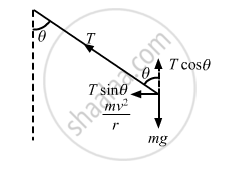Share

# A Simple Pendulum is Suspended from the Ceiling of a Car Taking a Turn of Radius 10 M at a Speed of 36 Km/H. Find the Angle Made by He String of the Pendulum with the Vertical - Physics

ConceptCircular Motion

#### Question

A simple pendulum is suspended from the ceiling of a car taking a turn of radius 10 m at a speed of 36 km/h. Find the angle made by he string of the pendulum with the vertical if this angle does not change during the turn. Take g = 10 m/s2.

#### Solution

Speed of the car = v = 36 km/hr = 10 m/s
Acceleration due to gravity = g = 10 m/sLet T be the tension in the string when the pendulum makes an angle θ with the vertical.
From the figure, we get :

$\text{T}\sin\theta = \frac{\text{mv}^2}{r} . . . (\text{i})$

$T\cos\theta = \text{mg . . . (ii)}$

$\Rightarrow \frac{\sin\theta}{\cos\theta} = \frac{\text{mv}^2}{\text{rmg}}$

$\Rightarrow \tan\theta = \frac{\text{v}^2}{\text{rg}}$

$\Rightarrow \theta = \tan^{- 1} \left( \frac{\text{v}^2}{\text{rg}} \right)$

$= \tan^{- 1} \left[ \frac{100}{(10 \times 10)} \right]$

$= \tan^{- 1} (1)$

$\Rightarrow \theta= 45^\circ$

Is there an error in this question or solution?

#### APPEARS IN

Solution A Simple Pendulum is Suspended from the Ceiling of a Car Taking a Turn of Radius 10 M at a Speed of 36 Km/H. Find the Angle Made by He String of the Pendulum with the Vertical Concept: Circular Motion.
S